## Collision Resolution Techniques-

In Hashing, collision resolution techniques are classified as-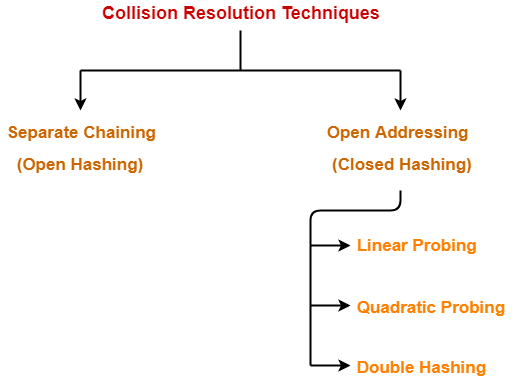In this article, we will compare separate chaining and open addressing.

## Separate Chaining Vs Open Addressing-

### Open Addressing

Keys are stored inside the hash table as well as outside the hash table. All the keys are stored only inside the hash table.

No key is present outside the hash table.

The number of keys to be stored in the hash table can even exceed the size of the hash table. The number of keys to be stored in the hash table can never exceed the size of the hash table.
Deletion is easier. Deletion is difficult.
Extra space is required for the pointers to store the keys outside the hash table. No extra space is required.

Cache performance is poor.

This is because of linked lists which store the keys outside the hash table.

Cache performance is better.

This is because here no linked lists are used.

Some buckets of the hash table are never used which leads to wastage of space.

Buckets may be used even if no key maps to those particular buckets.

## Which is the Preferred Technique?

The performance of both the techniques depend on the kind of operations that are required to be performed on the keys stored in the hash table-

## Separate Chaining-

Separate Chaining is advantageous when it is required to perform all the following operations on the keys stored in the hash table-

• Insertion Operation
• Deletion Operation
• Searching Operation

## NOTE-

• Deletion is easier in separate chaining.
• This is because deleting a key from the hash table does not affect the other keys stored in the hash table.

## Open Addressing-

Open addressing is advantageous when it is required to perform only the following operations on the keys stored in the hash table-

• Insertion Operation
• Searching Operation

## NOTE-

• Deletion is difficult in open addressing.
• This is because deleting a key from the hash table requires some extra efforts.
• After deleting a key, certain keys have to be rearranged.

To gain better understanding about Separate Chaining Vs Open Addressing,

Watch this Video Lecture

Get more notes and other study material of Data Structures.

Watch video lectures by visiting our YouTube channel LearnVidFun.

## Collision Resolution Techniques-

Before you go through this article, make sure that you have gone through the previous article on Collision Resolution Techniques.

We have discussed-

• Hashing is a well-known searching technique.
• Collision occurs when hash value of the new key maps to an occupied bucket of the hash table.
• Collision resolution techniques are classified as-In this article, we will discuss about Open Addressing.

## Open Addressing-

In open addressing,

• Unlike separate chaining, all the keys are stored inside the hash table.
• No key is stored outside the hash table.

Techniques used for open addressing are-

• Linear Probing
• Quadratic Probing
• Double Hashing

## Operations in Open Addressing-

Let us discuss how operations are performed in open addressing-

### Insert Operation-

• Hash function is used to compute the hash value for a key to be inserted.
• Hash value is then used as an index to store the key in the hash table.

In case of collision,

• Probing is performed until an empty bucket is found.
• Once an empty bucket is found, the key is inserted.
• Probing is performed in accordance with the technique used for open addressing.

### Search Operation-

To search any particular key,

• Its hash value is obtained using the hash function used.
• Using the hash value, that bucket of the hash table is checked.
• If the required key is found, the key is searched.
• Otherwise, the subsequent buckets are checked until the required key or an empty bucket is found.
• The empty bucket indicates that the key is not present in the hash table.

### Delete Operation-

• The key is first searched and then deleted.
• After deleting the key, that particular bucket is marked as “deleted”.

## NOTE-

• During insertion, the buckets marked as “deleted” are treated like any other empty bucket.
• During searching, the search is not terminated on encountering the bucket marked as “deleted”.
• The search terminates only after the required key or an empty bucket is found.

## Open Addressing Techniques-

Techniques used for open addressing are-

## 1. Linear Probing-

In linear probing,

• When collision occurs, we linearly probe for the next bucket.
• We keep probing until an empty bucket is found.

### Advantage-

• It is easy to compute.

### Disadvantage-

• The main problem with linear probing is clustering.
• Many consecutive elements form groups.
• Then, it takes time to search an element or to find an empty bucket.

### Time Complexity-

 Worst time to search an element in linear probing is O (table size).

This is because-

• Even if there is only one element present and all other elements are deleted.
• Then, “deleted” markers present in the hash table makes search the entire table.

## 2. Quadratic Probing-

In quadratic probing,

• When collision occurs, we probe for i2‘th bucket in ith iteration.
• We keep probing until an empty bucket is found.

## 3. Double Hashing-

In double hashing,

• We use another hash function hash2(x) and look for i * hash2(x) bucket in ith iteration.
• It requires more computation time as two hash functions need to be computed.

## Comparison of Open Addressing Techniques-

Yes No No
Yes Yes No
m m m2
##### Cache performance
Best Lies between the two Poor

### Conclusions-

• Linear Probing has the best cache performance but suffers from clustering.
• Quadratic probing lies between the two in terms of cache performance and clustering.
• Double caching has poor cache performance but no clustering.

## Load Factor (α)-

Load factor (α) is defined as-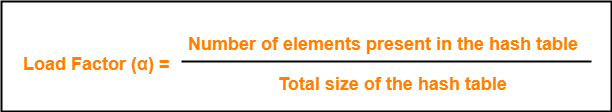In open addressing, the value of load factor always lie between 0 and 1.

This is because-

• In open addressing, all the keys are stored inside the hash table.
• So, size of the table is always greater or at least equal to the number of keys stored in the table.

## Problem-

Using the hash function ‘key mod 7’, insert the following sequence of keys in the hash table-

50, 700, 76, 85, 92, 73 and 101

Use linear probing technique for collision resolution.

## Solution-

The given sequence of keys will be inserted in the hash table as-

### Step-01:

• Draw an empty hash table.
• For the given hash function, the possible range of hash values is [0, 6].
• So, draw an empty hash table consisting of 7 buckets as-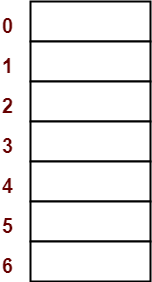### Step-02:

• Insert the given keys in the hash table one by one.
• The first key to be inserted in the hash table = 50.
• Bucket of the hash table to which key 50 maps = 50 mod 7 = 1.
• So, key 50 will be inserted in bucket-1 of the hash table as-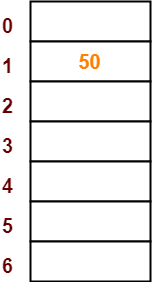### Step-03:

• The next key to be inserted in the hash table = 700.
• Bucket of the hash table to which key 700 maps = 700 mod 7 = 0.
• So, key 700 will be inserted in bucket-0 of the hash table as-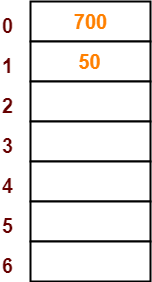### Step-04:

• The next key to be inserted in the hash table = 76.
• Bucket of the hash table to which key 76 maps = 76 mod 7 = 6.
• So, key 76 will be inserted in bucket-6 of the hash table as-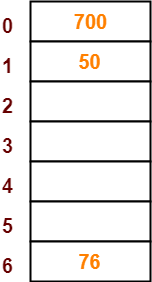### Step-05:

• The next key to be inserted in the hash table = 85.
• Bucket of the hash table to which key 85 maps = 85 mod 7 = 1.
• Since bucket-1 is already occupied, so collision occurs.
• To handle the collision, linear probing technique keeps probing linearly until an empty bucket is found.
• The first empty bucket is bucket-2.
• So, key 85 will be inserted in bucket-2 of the hash table as-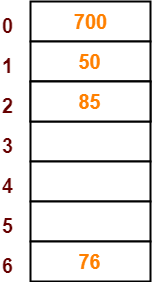### Step-06:

• The next key to be inserted in the hash table = 92.
• Bucket of the hash table to which key 92 maps = 92 mod 7 = 1.
• Since bucket-1 is already occupied, so collision occurs.
• To handle the collision, linear probing technique keeps probing linearly until an empty bucket is found.
• The first empty bucket is bucket-3.
• So, key 92 will be inserted in bucket-3 of the hash table as-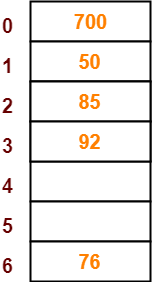### Step-07:

• The next key to be inserted in the hash table = 73.
• Bucket of the hash table to which key 73 maps = 73 mod 7 = 3.
• Since bucket-3 is already occupied, so collision occurs.
• To handle the collision, linear probing technique keeps probing linearly until an empty bucket is found.
• The first empty bucket is bucket-4.
• So, key 73 will be inserted in bucket-4 of the hash table as-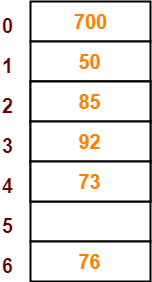### Step-08:

• The next key to be inserted in the hash table = 101.
• Bucket of the hash table to which key 101 maps = 101 mod 7 = 3.
• Since bucket-3 is already occupied, so collision occurs.
• To handle the collision, linear probing technique keeps probing linearly until an empty bucket is found.
• The first empty bucket is bucket-5.
• So, key 101 will be inserted in bucket-5 of the hash table as-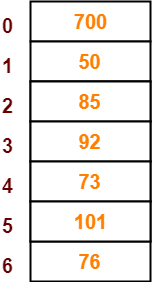To gain better understanding about Open Addressing,

Watch this Video Lecture

Next Article- Separate Chaining Vs Open Addressing

Get more notes and other study material of Data Structures.

Watch video lectures by visiting our YouTube channel LearnVidFun.

## Hashing in Data Structure-

Before you go through this article, make sure that you have gone through the previous article on Hashing.

We have discussed-

• Hashing is a well-known searching technique.
• It minimizes the number of comparisons while performing the search.
• It completes the search with constant time complexity O(1).

In this article, we will discuss about Collisions in Hashing.

## Collision in Hashing-

In hashing,

• Hash function is used to compute the hash value for a key.
• Hash value is then used as an index to store the key in the hash table.
• Hash function may return the same hash value for two or more keys.

 When the hash value of a key maps to an already occupied bucket of the hash table, it is called as a Collision.

## Collision Resolution Techniques-

 Collision Resolution Techniques are the techniques used for resolving or handling the collision.

Collision resolution techniques are classified as-1. Separate Chaining
2. Open Addressing

In this article, we will discuss about separate chaining.

## Separate Chaining-

To handle the collision,

• This technique creates a linked list to the slot for which collision occurs.
• The new key is then inserted in the linked list.
• These linked lists to the slots appear like chains.
• That is why, this technique is called as separate chaining.

## Time Complexity-

### For Searching-

• In worst case, all the keys might map to the same bucket of the hash table.
• In such a case, all the keys will be present in a single linked list.
• Sequential search will have to be performed on the linked list to perform the search.
• So, time taken for searching in worst case is O(n).

### For Deletion-

• In worst case, the key might have to be searched first and then deleted.
• In worst case, time taken for searching is O(n).
• So, time taken for deletion in worst case is O(n).

## Load Factor (α)-

Load factor (α) is defined as-If Load factor (α) = constant, then time complexity of Insert, Search, Delete = Θ(1)

## Problem-

Using the hash function ‘key mod 7’, insert the following sequence of keys in the hash table-

50, 700, 76, 85, 92, 73 and 101

Use separate chaining technique for collision resolution.

## Solution-

The given sequence of keys will be inserted in the hash table as-

### Step-01:

• Draw an empty hash table.
• For the given hash function, the possible range of hash values is [0, 6].
• So, draw an empty hash table consisting of 7 buckets as-### Step-02:

• Insert the given keys in the hash table one by one.
• The first key to be inserted in the hash table = 50.
• Bucket of the hash table to which key 50 maps = 50 mod 7 = 1.
• So, key 50 will be inserted in bucket-1 of the hash table as-### Step-03:

• The next key to be inserted in the hash table = 700.
• Bucket of the hash table to which key 700 maps = 700 mod 7 = 0.
• So, key 700 will be inserted in bucket-0 of the hash table as-### Step-04:

• The next key to be inserted in the hash table = 76.
• Bucket of the hash table to which key 76 maps = 76 mod 7 = 6.
• So, key 76 will be inserted in bucket-6 of the hash table as-### Step-05:

• The next key to be inserted in the hash table = 85.
• Bucket of the hash table to which key 85 maps = 85 mod 7 = 1.
• Since bucket-1 is already occupied, so collision occurs.
• Separate chaining handles the collision by creating a linked list to bucket-1.
• So, key 85 will be inserted in bucket-1 of the hash table as-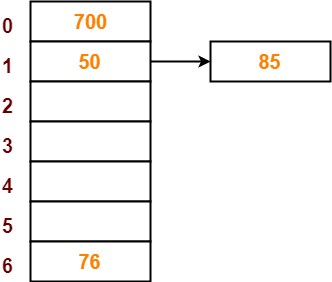### Step-06:

• The next key to be inserted in the hash table = 92.
• Bucket of the hash table to which key 92 maps = 92 mod 7 = 1.
• Since bucket-1 is already occupied, so collision occurs.
• Separate chaining handles the collision by creating a linked list to bucket-1.
• So, key 92 will be inserted in bucket-1 of the hash table as-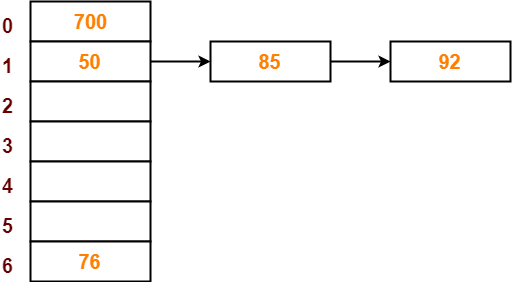### Step-07:

• The next key to be inserted in the hash table = 73.
• Bucket of the hash table to which key 73 maps = 73 mod 7 = 3.
• So, key 73 will be inserted in bucket-3 of the hash table as-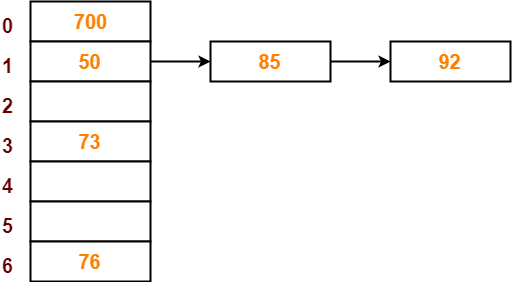### Step-08:

• The next key to be inserted in the hash table = 101.
• Bucket of the hash table to which key 101 maps = 101 mod 7 = 3.
• Since bucket-3 is already occupied, so collision occurs.
• Separate chaining handles the collision by creating a linked list to bucket-3.
• So, key 101 will be inserted in bucket-3 of the hash table as-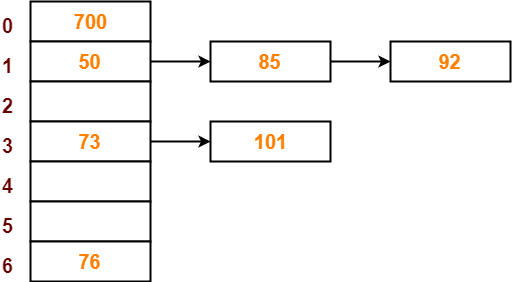To gain better understanding about Separate Chaining,

Watch this Video Lecture

Next Article- Open Addressing

Get more notes and other study material of Data Structures.

Watch video lectures by visiting our YouTube channel LearnVidFun.

## Searching Techniques-

In data structures,

• There are several searching techniques like linear search, binary search, search trees etc.
• In these techniques, time taken to search any particular element depends on the total number of elements.

### Example-

• Linear Search takes O(n) time to perform the search in unsorted arrays consisting of n elements.
• Binary Search takes O(logn) time to perform the search in sorted arrays consisting of n elements.
• It takes O(logn) time to perform the search in Binary Search Tree consisting of n elements.

### Drawback-

The main drawback of these techniques is-

• As the number of elements increases, time taken to perform the search also increases.
• This becomes problematic when total number of elements become too large.

## Hashing in Data Structure-

In data structures,

• Hashing is a well-known technique to search any particular element among several elements.
• It minimizes the number of comparisons while performing the search.

### Advantage-

Unlike other searching techniques,

• Hashing is extremely efficient.
• The time taken by it to perform the search does not depend upon the total number of elements.
• It completes the search with constant time complexity O(1).

## Hashing Mechanism-

In hashing,

• An array data structure called as Hash table is used to store the data items.
• Based on the hash key value, data items are inserted into the hash table.

## Hash Key Value-

• Hash key value is a special value that serves as an index for a data item.
• It indicates where the data item should be be stored in the hash table.
• Hash key value is generated using a hash function.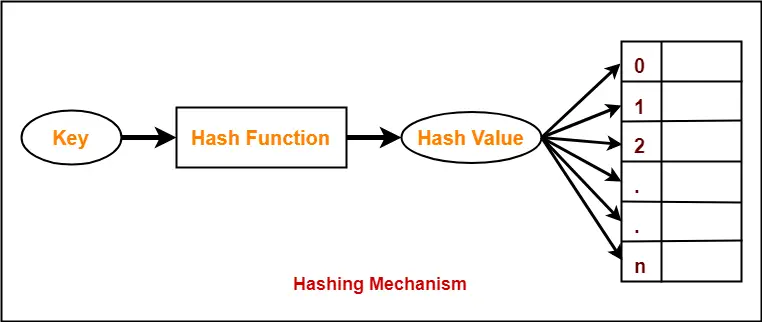## Hash Function-

 Hash function is a function that maps any big number or string to a small integer value.

• Hash function takes the data item as an input and returns a small integer value as an output.
• The small integer value is called as a hash value.
• Hash value of the data item is then used as an index for storing it into the hash table.

## Types of Hash Functions-

There are various types of hash functions available such as-

1. Mid Square Hash Function
2. Division Hash Function
3. Folding Hash Function etc

It depends on the user which hash function he wants to use.

## Properties of Hash Function-

The properties of a good hash function are-

• It is efficiently computable.
• It minimizes the number of collisions.
• It distributes the keys uniformly over the table.

To gain better understanding about Hashing in Data Structures,

Watch this Video Lecture

Next Article- Collision in Hashing

Get more notes and other study material of Data Structures.

Watch video lectures by visiting our YouTube channel LearnVidFun.